U.S. Geological Survey Data Series 311

# Seismic Profiles

#### Home | Acronyms | Contents | Navigation | Profiles | Logs | Metadata | Software

Below are thumbnail images of each line with links to full-size printable and interactive seismic reflection profiles. The printable profiles are GIF images that were gained using Seismic Unix software. These images are stored in the PRINTABLE folder. Refer to the Software page for details about the processing and examples of the processing scripts.

The processed SEG-Y data were then exported to CTI SonarWeb software to produce interactive versions of the profiles that allow the user to obtain a geographic location and depth from the profile for a cursor position. This information is displayed in the status bar of the browser. The interactive profiles, which are stored in the INTERACTIVE folder, have been corrected for the offset between the shotpoint and GPS antenna (see fig. 1).

Table 2 lists trackline statistics for 06SCC03.

NOTE: The only supported Web browsers that properly display all features of the interactive profiles are Internet Explorer 6 for Windows and Firefox 1.5 for all platforms. Use of other browsers may result in spurious or no information given in the status window. Firefox may be downloaded at http://www.mozilla.org/products/firefox.

Browser setup for viewing interactive profiles:
For all browsers, the status bar and JavaScript must be enabled. Firefox also requires "Change status bar text" to be checked under Advanced JavaScript Options.

NOTE: Due to a malfunction with the acquistion software, incorrect navigation data were written to the SEG-Y headers for most of line 06c01; therefore, the interactive profile created for this line does not show actual navigation fixes observed during data collection.

NOTE: Depths shown are relative to the depth of the towfish, not to sea level

SEG-Y Format

Line: 06c01 16691 traces:
Start of line:   06c01     SHOT=1 day=199 hour=14   minute=5  second=32
End of line:   06c01 SHOT=16691 day=199 hour=15  minute=15  second=50
INTERACTIVE | PRINTABLE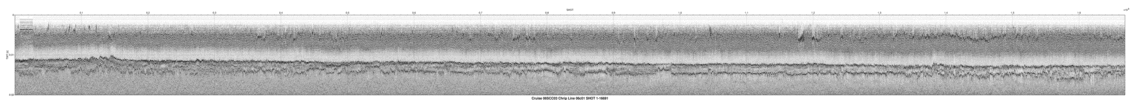Line: 06c02_a  9999 traces:
Start of line: 06c02_a     SHOT=1 day=199 hour=15  minute=15  second=52
End of line: 06c02_a  SHOT=9999 day=199 hour=15  minute=57  second=39
INTERACTIVE | PRINTABLE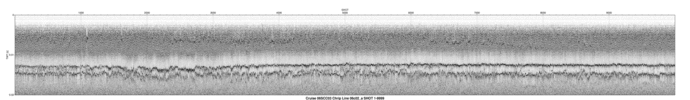Line: 06c02_b 12603 traces:
Start of line: 06c02_b SHOT=10000 day=199 hour=15  minute=57  second=39
End of line: 06c02_b SHOT=22602 day=199 hour=16  minute=50   second=0
INTERACTIVE | PRINTABLE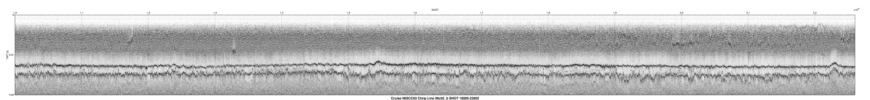Line: 06c03  3903 traces:
Start of line:   06c03     SHOT=1 day=199 hour=16  minute=50   second=4
End of line:   06c03  SHOT=3903 day=199 hour=17   minute=6  second=32
INTERACTIVE | PRINTABLE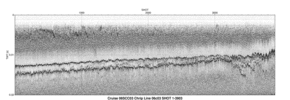Line: 06c04_a  9999 traces:
Start of line: 06c04_a     SHOT=1 day=199 hour=17   minute=6  second=34
End of line: 06c04_a  SHOT=9999 day=199 hour=17  minute=48  second=19
INTERACTIVE | PRINTABLE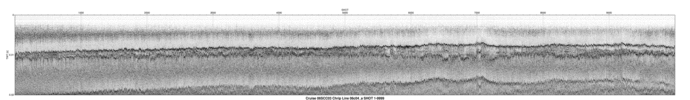Line: 06c04_b 18972 traces:
Start of line: 06c04_b SHOT=10000 day=199 hour=17  minute=48  second=19
End of line: 06c04_b SHOT=28971 day=199 hour=19   minute=7   second=8
INTERACTIVE | PRINTABLE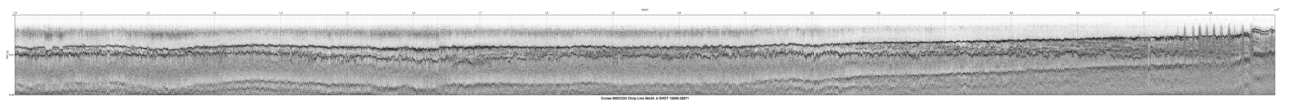Line: 06c05  5517 traces:
Start of line:   06c05     SHOT=1 day=199 hour=19   minute=7  second=10
End of line:   06c05  SHOT=5517 day=199 hour=19  minute=30  second=19
INTERACTIVE | PRINTABLE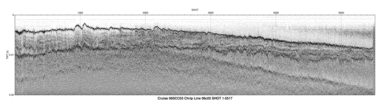Line: 06c06  6125 traces:
Start of line:   06c06     SHOT=1 day=199 hour=19  minute=30  second=37
End of line:   06c06  SHOT=6125 day=199 hour=19  minute=56  second=15
INTERACTIVE | PRINTABLE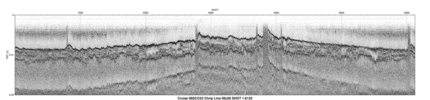Line: 06c07  8531 traces:
Start of line:   06c07     SHOT=1 day=199 hour=19  minute=56  second=19
End of line:   06c07  SHOT=8531 day=199 hour=20  minute=31  second=57
INTERACTIVE | PRINTABLE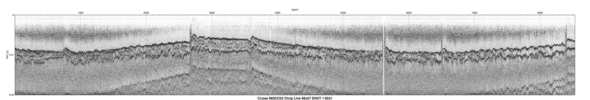Line: 06c08  8398 traces:
Start of line:   06c08     SHOT=1 day=199 hour=20  minute=31  second=59
End of line:   06c08  SHOT=8398 day=199 hour=21   minute=7   second=5
INTERACTIVE | PRINTABLE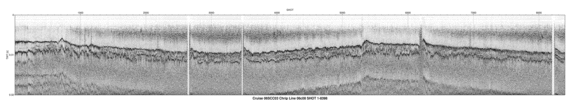Line: 06c09  6404 traces:
Start of line:   06c09     SHOT=1 day=199 hour=21   minute=7   second=7
End of line:   06c09  SHOT=6404 day=199 hour=21  minute=33  second=52
INTERACTIVE | PRINTABLE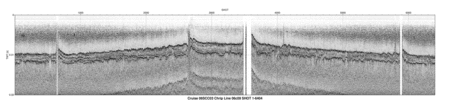Line: 06c10  5197 traces:
Start of line:   06c10     SHOT=1 day=199 hour=21  minute=33  second=54
End of line:   06c10  SHOT=5197 day=199 hour=21  minute=55  second=42
INTERACTIVE | PRINTABLE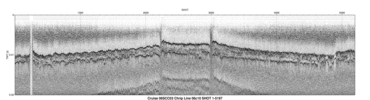Line: 06c11  2846 traces:
Start of line:   06c11     SHOT=1 day=200 hour=16  minute=15  second=20
End of line:   06c11  SHOT=2846 day=200 hour=16  minute=27  second=27
INTERACTIVE | PRINTABLE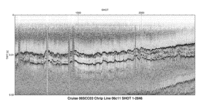Line: 06c12  3162 traces:
Start of line:   06c12     SHOT=1 day=200 hour=16  minute=27  second=32
End of line:   06c12  SHOT=3162 day=200 hour=16  minute=40  second=51
INTERACTIVE | PRINTABLE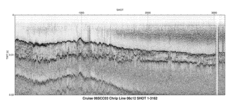Line: 06c13  2798 traces:
Start of line:   06c13     SHOT=1 day=200 hour=16  minute=40  second=53
End of line:   06c13  SHOT=2798 day=200 hour=16  minute=52  second=41
INTERACTIVE | PRINTABLE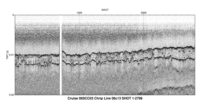Line: 06c14  3026 traces:
Start of line:   06c14     SHOT=1 day=200 hour=16  minute=52  second=43
End of line:   06c14  SHOT=3026 day=200 hour=17   minute=5  second=26
INTERACTIVE | PRINTABLE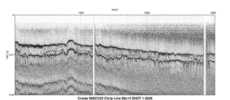Line: 06c15  2899 traces:
Start of line:   06c15     SHOT=1 day=200 hour=17   minute=5  second=28
End of line:   06c15  SHOT=2899 day=200 hour=17  minute=17  second=37
INTERACTIVE | PRINTABLE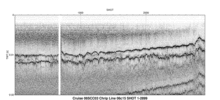Line: 06c16  3130 traces:
Start of line:   06c16     SHOT=1 day=200 hour=17  minute=17  second=38
End of line:   06c16  SHOT=3130 day=200 hour=17  minute=30  second=46
INTERACTIVE | PRINTABLE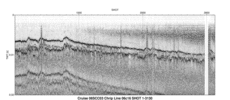Line: 06c17  2687 traces:
Start of line:   06c17     SHOT=1 day=200 hour=17  minute=30  second=47
End of line:   06c17  SHOT=2687 day=200 hour=17  minute=42   second=4
INTERACTIVE | PRINTABLE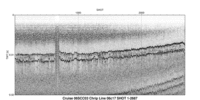Line: 06c18  2963 traces:
Start of line:   06c18     SHOT=1 day=200 hour=17  minute=42   second=5
End of line:   06c18  SHOT=2963 day=200 hour=17  minute=54  second=37
INTERACTIVE | PRINTABLE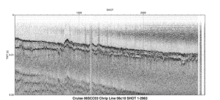Line: 06c19  2748 traces:
Start of line:   06c19     SHOT=1 day=200 hour=17  minute=54  second=40
End of line:   06c19  SHOT=2748 day=200 hour=18   minute=6  second=16
INTERACTIVE | PRINTABLE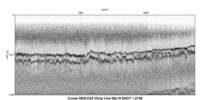Line: 06c20  5219 traces:
Start of line:   06c20     SHOT=1 day=200 hour=18   minute=7   second=0
End of line:   06c20  SHOT=5219 day=200 hour=18  minute=28  second=50
INTERACTIVE | PRINTABLE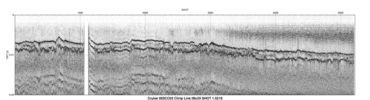Line: 06c21  3086 traces:
Start of line:   06c21     SHOT=1 day=200 hour=18  minute=29  second=12
End of line:   06c21  SHOT=3086 day=200 hour=18  minute=42  second=17
INTERACTIVE | PRINTABLE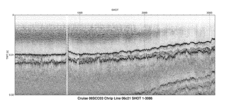Line: 06c22_a  9999 traces:
Start of line: 06c22_a     SHOT=1 day=200 hour=18  minute=44  second=37
End of line: 06c22_a  SHOT=9999 day=200 hour=19  minute=26  second=22
INTERACTIVE | PRINTABLE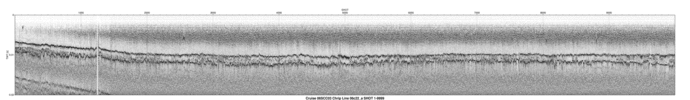Line: 06c22_b 16662 traces:
Start of line: 06c22_b SHOT=10000 day=200 hour=19  minute=26  second=22
End of line: 06c22_b SHOT=26661 day=200 hour=20  minute=35  second=31
INTERACTIVE | PRINTABLE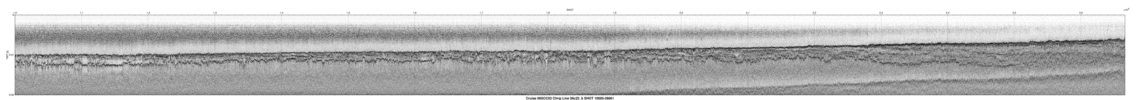#### Home | Acronyms | Contents | Navigation | Profiles | Logs | Metadata | Software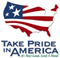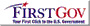U.S. Department of the Interior | U.S. Geological Survey
URL: pubsdata.usgs.gov/pubs/ds/311/html/profiles.html
Page Contact Information: Publishing Services